The K5 Learning Blog urges parents to be pro-active in helping their children reach their full academic potential.

K5 Learning
provides free worksheets, workbooks and an online reading and math program for kindergarten to grade 5 students.Fractions are parts of a whole.  To understand fractions we need to understand each part of the fraction.

The bottom number is called the denominator. The denominator tells us two things:

1.    How many parts our whole is divided into;
2.    What to call these parts.

For example in the fraction 2/3: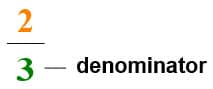This fraction is divided into three parts and it’s called “third”. In this case it’s two thirds.

The top number is called the numerator.  It shows the number of those parts we have.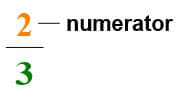This tells us that we have 2 out of 3 equal parts.

## How to add fractions with like denominators

There are three simple steps to add simple fractions:

1.    Make sure the bottom numbers (denominators) are the same. For example: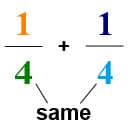2.    Add the top numbers (numerators):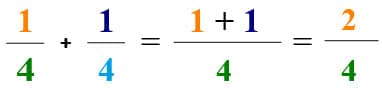3.    Simplify the fraction (if needed):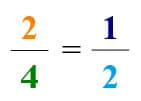## Simplifying fractions

It’s important to give your answers in the simplest form. You need to make sure your solution is reduced to its lowest terms/numbers.

Let’s look at this example: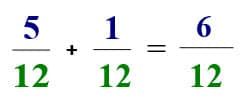To do this:

1.    Consider the factors of the numerator and denominator

2.    Think about the Greatest Common Factor: the highest number that divides evenly into both the numerator and the denominator.

6 divides into 1, 2, 3, 6
12 divides into 1, 2, 3, 4, 6, 12

The Greatest Common Factor for the numerator and denominator is 6. So: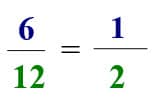As 6 divided by 6 is 1, and 12 divided by 6 is 2.

For practice, try our grade 3 fractions worksheets.

In our next post, we’ll tackle how to add fractions with unlike denominators.